Study Guide

# Factors and Roots of Polynomials

## Factors and Roots of Polynomials

We're having a blast here on the planet of polynomials. Our little friends have been very hospitable. We've been getting quite a workout adding, subtracting, and multiplying.

We're nearly ready to start our trek up Polynomial Mountain. We have one final preparation to make: we'll need a technique called synthetic division. Once we have it, we'll set out to find a new trick called the Remainder Theorem. It'll show us the how to find factors and roots of polynomials. It also loves to draw squiggly little lines in the sand. We can't wait to play in the sand.

• ### Synthetic Division

We're starting to get impatient here. We want to start climbing Polynomial Mountain, but we won't be able to make it to the top without synthetic division, a fancier type of polynomial division. Maybe it's synthetic because it's made out of some space-age polymer?

### Sample Problem

Divide x2 + 8x + 15 by x + 3.

To start off, we strip away all of our variables from our polynomial and put the numbers into an open box, like so: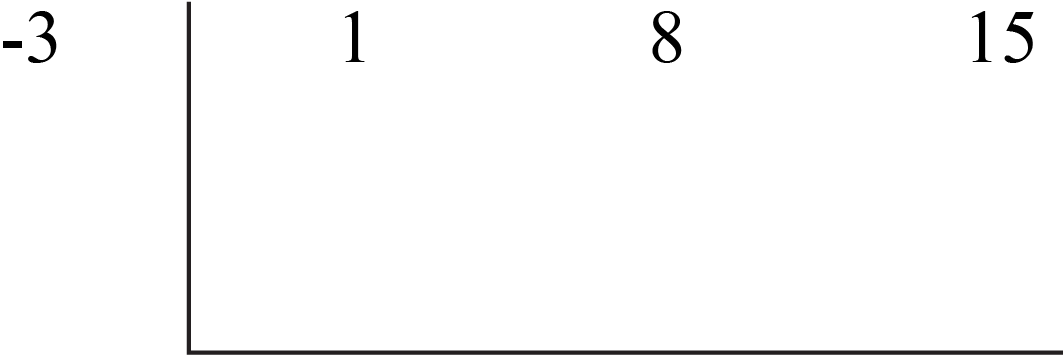Hey, wait a second. That -3 isn't from the polynomial, and it isn't in the box. What gives?

In synthetic division, we're always dividing a polynomial by xa. That's where the -3 came from; it's a. We're dividing by x + 3, which is the same thing as x – (-3). Now we can make the division happen.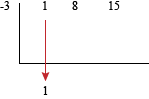Step one is to drop the first coefficient (from 1x2) below the line. Next we'll multiply that 1 by a, -3, and put it into the second column.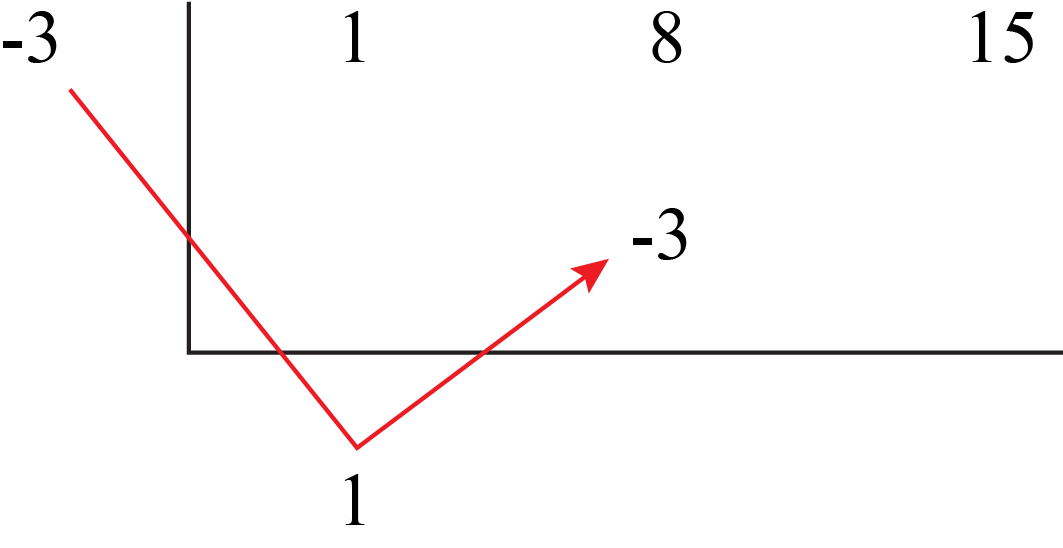We get another -3. We'd better hurry before they start multiplying out of control. We'll add it to the 8 already in that column, and put the result down below.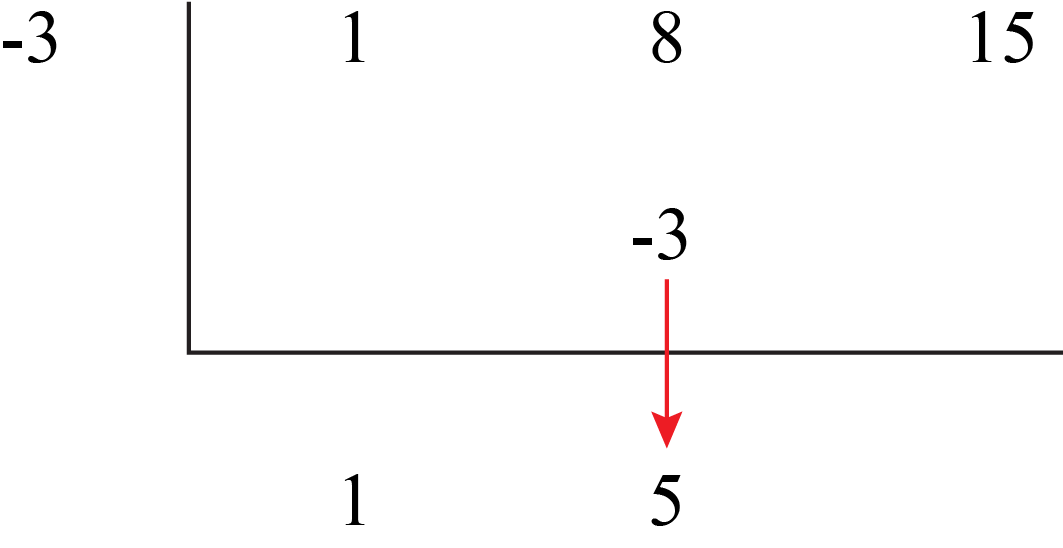Now we just keep going like we did before: multiply the number at the bottom by our -3, and then add the result to the next column. We'll do this until we run out of numbers.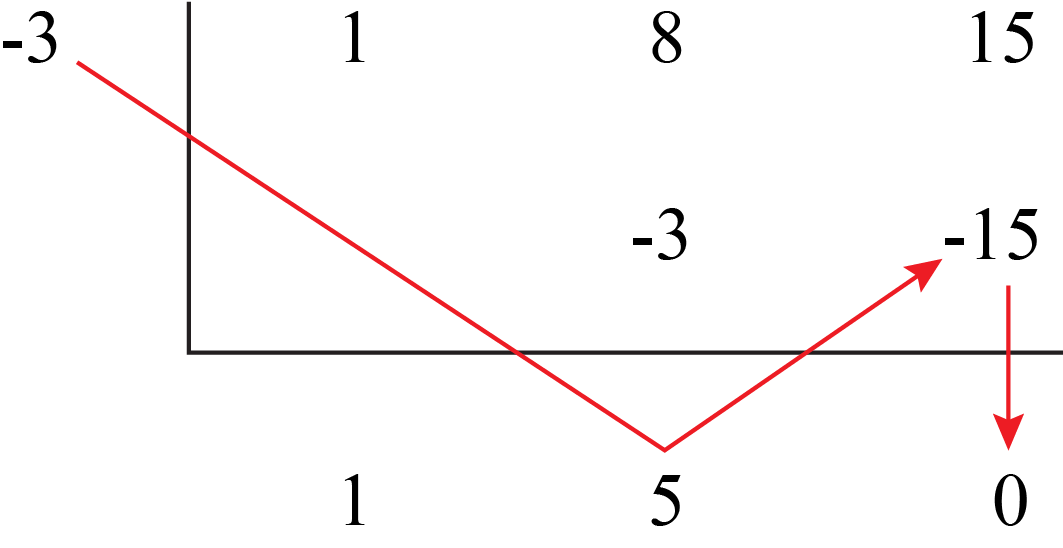All gone already? Oh well. Anyway, the rightmost number below the line is the remainder from our division. It's 0, so we have no remainder. That means that x + 3 is a factor of x2 + 8x + 15, since it divides evenly.

Our leftover numbers below the box give us the answer to the division problem. From the right, they start with the remainder, then the constant, in this case 5, and increase in degree as we move left. That means that we have x + 5 as our quotient.

Our final answer is that x2 + 8x + 15 = (x + 3)(x + 5). No, wait, our final answer is (D) a jar of mayonnaise. Did we win, Regis? Did we? Are we going to be a millionaire?

Oh. No. We were right before.

One quick note before we finish up. If our polynomial skips over any powers of x, we need to use a 0 as a placeholder in our synthetic division. For example if we were dividing x3 + 2x + 50 by x + 4, the top line of our synthetic division box would be 1, 0, 2, 50. That's because our polynomial skipped the x2 term, so we're actually dividing x3 + 0x2 + 2x + 50. Got it?

That was the last thing we needed to do before starting up Polynomial Mountain. We hope it goes smoother than a climb of Mount Everest.

• ### The Remainder Theorem

We just started hiking up Polynomial Mountain, and we've already found it. The Remainder Theorem.

No worries—we know its name sounds scary. We hear "remainder" and think "division" and shudder, but this is actually another little trick showing us how to evaluate polynomials for a specific value. And we love little tricks—right?More good news: if atmospheric conditions are right, this is also a way to avoid long division, and a whole lot of headaches altogether.

Hang on. Our polynomial friends are clearing their throats. They want to say something to us.

If we divide a polynomial, P(x), by xa, then the remainder equals P(a).

That must be it: the secret of The Remainder Theorem. They've entrusted it to us. Let's give it a whirl.

### Sample Problem

Find the remainder when 3x2 – 4x + 5 is divided by x – 1.

According to our brainy polynomial friends, we need to plug x = 1 into our expression. Remember, we're dividing by xa, so we need 1, not -1. Using the wrong sign would teleport our answer straight to Wrongville. Not good.

P(1) = 3(1)2 – 4(1) + 5

= 3 – 4 + 5

= 4

The remainder is 4. Now we know that x – 1 does not divide evenly into 3x2 – 4x + 5.

The polynomials are chittering away and getting excited. They want to show us another example. We'd hate to disappoint them.

### Sample Problem

Find the remainder when 2x2 + x – 7 is divided by x + 2.

We start by plugging -2 into our polynomial.

P(-2) = 2(-2)2 + (-2) – 7

= 2(4) – 2 – 7

= 8 – 9 = -1

Okay, the remainder is -1. This isn't too bad.

Uh oh. They're bringing us another problem to solve. They're really into this. We can do one more, but that's it.

### Sample Problem

Find the remainder when 2x3 – 2x2 – 2x + 2 is divided by x – 3.

We like this polynomial, so we're gonna go ahead and stick a 3 on it.

P(3) = 2(3)3 – 2(3)2 – 2(3) + 2

= 2(27) – 2(9) – 6 + 2

= 54 – 18 – 4

= 32

Okay, the remainder is 32, and we're done now. That's the end of—wait, they're bringing another problem. Make a break for it.

• ### Finding Factors and Roots of Polynomials

All right, we've trekked a little further up Polynomial Mountain and have come to another impasse. Now we've gotta find factors and roots of polynomials.

Hey, our polynomial buddies have caught up to us, and they seem to have calmed down a bit. They have a polynomial for us.

2x3 + x2 – 2x – 1

We start with our new discovery, the Remainder Theorem. It'll tell us if something is a factor of this polynomial. It can't tell us if we picked the right lottery numbers, though.

For instance, is x – 1 a factor? Is x – 1 one of the lotto numbers?

To answer the first question, we can plug x = 1 into the polynomial. If we get a 0, then x – 1 is a factor, because it divides the polynomial with no remainder. If we don't get 0, then it isn't.

P(1) = 2(1)3 + (1)2 – 2(1) – 1

= 2 + 1 – 2 – 1

= 0

Yep, this means that x – 1 is a factor of this polynomial. It tells us nothing about our chances for the Powerball. Good thing we didn't pin all our hopes on it.

Now what? Factors are multiplied together, so there must be at least one more factor left.

Our space-alien friends say we can use synthetic division to find the other factors.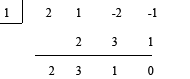We've gone ahead and laid it all out in one step. We can see that 2x3 + x2 – 2x – 1 divided by x – 1 is:

2x2 + 3x + 1

That's our other factor, but we can factor that a bit more to get:

2x2 + 3x + 1 =
(2x + 1)(x + 1)

This means our polynomial is actually equal to:

P(x) = (2x + 1)(x + 1)(x – 1)

Did you forget our first factor, x – 1? It's still there. We can now set each factor equal to zero to find the roots. That gives us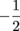, -1, and 1.

Excellent. That's everything we wanted to know, and all before teatime. How about we keep working our way up Polynomial Mountain with a few more problems?

### Sample Problem

See if x + 1 is a factor of x4 + x3 + 3x2x + 1.

We plug and chug away, by shoving x = -1 into the polynomial.

P(-1) = (-1)4 + (-1)3 + 3(-1)2 – (-1) + 1

= 1 – 1 + 3 + 1 + 1

= 5

End of the line, hoss. A 5 is definitely not a 0, so x + 1 is not a factor for this polynomial. It doesn't divide through evenly. Mosey on to the next problem.

### Sample Problem

Write a polynomial with the roots -3, 3, and 2.

Huh. Looks like we need to go backwards this time. Listen closely, and you might hear that Paul is dead.

If the roots are -3, 3, and 2, that means the factors are:

(x + 3), (x – 3), (x – 2)

Plug each root in for x, and you'll get zero, zero, zero every time. So our polynomial must be:

P(x) = (x + 3)(x – 3)(x – 2)

Which multiplies out to:

P(x) =(x2 – 9)(x – 2)

P(x) = x3 – 2x2 – 9x + 18

Wait, we just heard that Paul is, in fact, not dead. False alarm.

### Sample Problem

Find all roots of x3 + 3x2x – 3.

Now this is a little tougher situation. What do we do if no factors are given to us?

Well, magic has a long, if unreliable, history of use in cases like this. However, most people aren't 7th level clerics capable of casting Divination, so maybe we should try something else.

All of our factors take the form of xa. The a needs to multiply into the constant at the end of the polynomial, in this case -3. That means our factor (for the polynomial) needs a factor (from that constant).

The factors of the constant -3 are ±3 and ±1. We'll pick one and run it through the Remainder Theorem. A 3 sounds good. If P(3) = 0, then 3 is a root, which means x – 3 is a factor.

P(3) = (3)3 + 3(3)2 – (3) – 3

= 27 + 27 – 6

= 48

3 is not a root. Let's guess something else, like -3.

P(-3) = (-3)3 + 3(-3)2 – (-3) – 3

= -27 + 27 + 3 – 3

= 0

Okay. Step one: done. That means x + 3 is a factor. Now we can use synthetic division to find the other factors, and then all of the roots.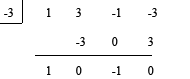That gives us x2 – 1 as our other factor. We can factor that factor to get (x + 1)(x – 1).

This means our polynomial is:

P(x) = (x + 3)(x + 1)(x – 1)

That, dear Shmooper, gives us roots of -3, -1, and 1.

We did it. We're so smart (not to brag, or anything like that).

### Sample Problem

Find all roots of x3 + 2x2 – 25x – 50, given that one root is 5.

If one root is 5, that means x – 5 is a factor. We can use synthetic division to find the rest.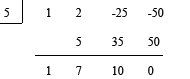Our other factor is x2 + 7x + 10. Further factoring furnishes fitting fanswers—er, answers.

(x + 2)(x + 5)

That means our full polynomial is:

P(x) = (x – 5)(x + 2)(x + 5)

The roots for this polynomial are x = 5, -2, and -5.

Finally. Good job, mathronaut. Onward and upward.

• ### Using Roots to Construct Rough Graphs of Polynomials

We're trekking right up Polynomial Mountain with our guides, the polynomials. What are those crazy little guys doing now? It looks like they're all drawing squiggly lines in the sand.

Wait. Are they writing their names or drawing a picture of themselves? That's it. When we look carefully, we see that they've used their roots to draw self-portraits in the sand. We can even see patterns based on their degree.

Could we use what we've discovered on the planet of polynomials to make our own sketches of our polynomial friends?

Yes. We think we're ready to construct some rough sketches of these guys. From our previous visit to this planet, we already know how to graph first- and second-degree polynomials (a.k.a. linear and quadratic functions). Now we'll look at how to use their roots to construct rough graphs of higher-order polynomials. We can use the Remainder Theorem to check for roots, and then use synthetic division and factoring to find the rest.Let's ask our alien friends for some of their little tricks and hints to make this easy.

First, the roots, also called the zeros, of our polynomials are the points where they cross the x-axis.

Second, we can have a general idea what our graph will look like based on the degree of the polynomial.

The two ends of an even-degree polynomial graph as either "up" on both ends or "down" on both ends. Like this: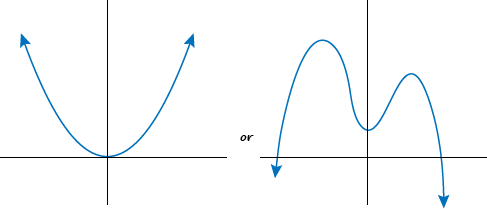Odd-degree polynomials graph with one end "up" and one end "down."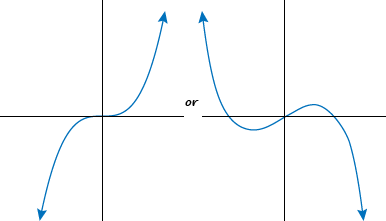We call all this a polynomial's end behavior. Polynomials don't see any difference between front-end behavior and rear-end behavior, which explains why they can be so hard to get along with sometimes.

The last hint we have as to the shape of our graph is the degree of the polynomial. The hint has an official name, the Turning Point Theorem, but we don't need to worry about that.

The basic idea is that a graph always has fewer turning points than its polynomial's degree.

The graph of a second-degree polynomial has at most 1 turn, while a third-degree polynomial has at most 2 turns. A turning point is a spot where the polynomial changes direction (moving up or down).

The best way to see how this all plays out is to actually start making a graph.

### Sample Problem

Graph y = x3 – 7x + 6.

First we'll find possible roots by looking at the factors of the constant 6. They are x = ±6, ±3, ±2, and ±1. Nothing could be simpler than plugging in a 1, so we'll do that first.

P(1) = (1)3 – 7(1) + 6

= 1 – 7 + 6

= 0

Whether it's efficiency or laziness, we won't argue with results: we know that x – 1 must be a factor of our polynomial, and one of its roots is x = 1. Now we use this 1 in our synthetic division to find all the factors of the polynomial.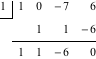The second factor is x2 + x – 6. Factoring again gives us (x + 3)(x – 2). Mix all our factors together to find the roots.

P(x) = (x – 1)(x + 3)(x – 2)

Setting each part equal to zero gives us roots of x = 1, -3, and 2. That means the points (1, 0), (-3, 0), and (2, 0) all show up on our graph.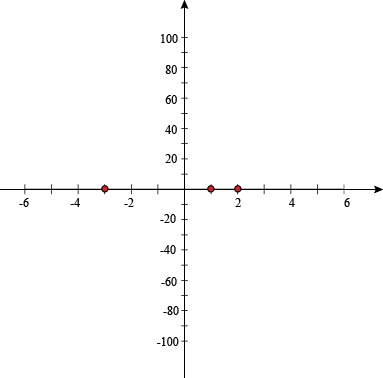Notice that the graph must touch the x-axis at these three points, and possibly cross over. Not in a "now we're dead" sense, but in a "now our sign is the opposite" one.

Our polynomial has degree 3 (because of x3), and this is odd. Not unusual; just odd. Its end behavior will have one end pointing up and one end pointing down.

We'll need at least one more point in each section of the graph to get the general shape. What are the sections? There's one on each side of each root.

We'll just pick a few x-values, each one from a different section of the graph.

P(-5) = (-5)3 – 7(-5) + 6 = -84 → (-5, -84)

P(0) = (0)3 – 7(0) + 6 = 6 → (0, 6)

P(1.5) = (1.5)3 – 7(1.5) + 6 = -1.125 → (1.5, -1.125)

P(5) = (5)3 – 7(5) + 6 = 96 → (5, 96)

Now we have enough points to get a rough graph of our polynomial, by plotting these additional four points and connecting them with a smooth curve. We can afford to be a bit loosey-goosey with our drawing. We just want to get the overall behavior of the polynomial.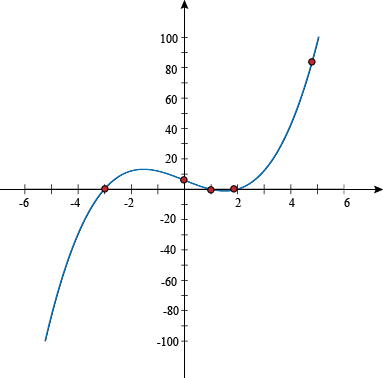Now we can see that the graphs turns twice, between x = -3 and 1, and between x = 1 and 2. This beautiful graph turns points and heads.

### Sample Problem

Graph f(x) = x3 + x2 – 22x – 40.

The first rule of graphing polynomials is, "Don't talk about graphing polynomials." Whoops, we already broke that one. The second rule is, "Find possible roots by looking at the factors of the constant." Following one out of two rules isn't that bad, right?

The factors of 40 are ±40, ±20, ±10, ±8, ±5, ±4, ±2 and ±1. That's a lot of factors. Dig in.

f(1) = (1)3 + (1)2 – 22(1) – 40

= 1 + 1 – 62

= -60

Nope, that isn't a factor. Plugging in -1 wouldn't improve things much, either. Let's try 2.

f(2) = (2)3 + (2)2 – 22(2) – 40

= 8 + 4 – 44 – 40

= -72

Swing and a miss. Maybe -2?

f(-2) = (-2)3 + (-2)2 – 22(-2) – 40

= -8 + 4 + 44 – 40

= 0

Ding, ding, ding, we have a winner. Our first root is x = -2, so our first factor is x + 2.

We run that -2 through a round of synthetic division to find our other roots.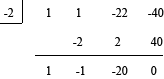Our polynomial is:

f(x) = (x + 2)(x2x – 20)

This factors further to:

f(x) = (x + 2)(x + 4)(x – 5)

Our roots, ladies and gents: x = -2, -4, and 5. Give them a round of applause as they take their spots on the graph.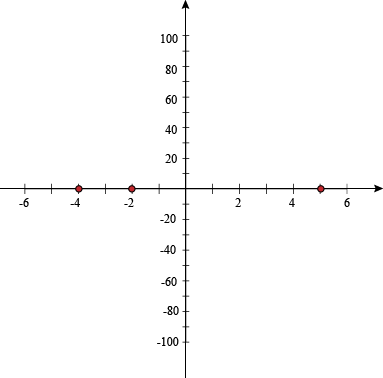We need more points, though. We'll pick a few more x-values to try, one from each section. Other numbers would work, but we want to keep the numbers small and manageable.

f(-5) = (-5)3 + (-5)2 – 22(-5) – 40 = -30 → (-5, -30)

f(-3) = (-3)3 + (-3)2 – 22(-3) – 40 = 8 → (-3, 8)

f(0) = (0)3 + (0)2 – 22(0) – 40 = -40 → (0, -40)

f(6) = (6)3 + (6)2 – 22(6) – 40 = 80 → (6, 80)

Now we have enough points to get a rough graph of our polynomial. We'll need even more points if we want to make the leaderboards, though.

The degree of the polynomial is odd, so it will have the two ends pointing in opposite directions.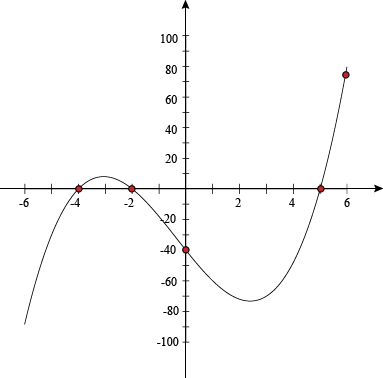That's an award-winning graph right there. For what award, we're not sure. Maybe the Most Recent Graph Award.

That isn't really a ringing endorsement, is it? Let's try another one.

### Sample Problem

Graph y = x5.

Um, we think we found a zero already. It's zero.

P(0) = (0)5 = 0

The degree, 5, is odd, so we know the end behavior has one end "up" and the other "down." It's everything in the middle that's kind of hazy.

We don't have any nice sections to choose points from, so we'll make a table and calculate several values to get our ordered pairs.Three numbers in each direction should be enough to have a rough idea of what the graph looks like, and a rough idea is all we need.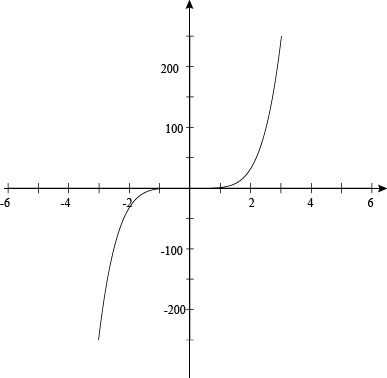Okay, that was our third odd graph in a row. We need to switch things up, and then we'll blow this popsicle stand.

### Sample Problem

Graph f(x) = x4 – 9x2.

With all the use we've gotten out of the Remainder Theorem to find factors and roots, we can forget that sometimes it's easier to just factor the polynomial directly.

x4 – 9x2 = 0

x2(x2 – 9) = 0

Our roots here are x = 0 and x = ± 3. See? Simple. Don't work for the math if you can avoid it. Make the math work for you.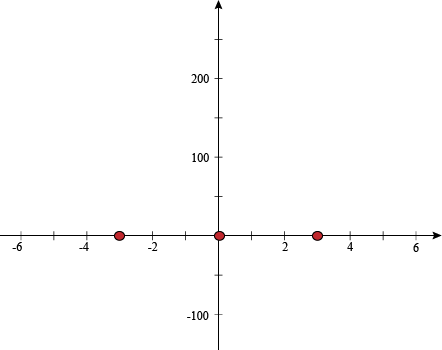Even-degree polynomials have end behavior where the ends both point up or both point down. Its degree is 4, so can have no more than 3 turns. Also, this is the last problem of the section, and we're tired, so it can't be too hard. We just need to find a few more points. Let's slice it up into sections again, and find one point between each of those roots.

P(-5) = (-5)4 – 9(-5)2 = 400 → (-5, 400)

P(-2) = (-2)4 – 9(-2)2 = -20 → (-2, -20)

P(2) = (2)4 – 9(2)2 = -20 → (2, -20)

P(5) = (5)4 – 9(5)2 = 400 → (-5, 400)

Wait, we could've plotted x = ±1 and ±4, and the numbers wouldn't have been as hard. That's what happens when graphing while tired, we suppose. That, and graphs that look suspiciously like beds, and sheep, and pillows.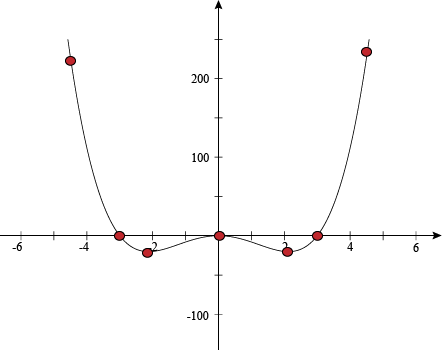All right, everything looks good here. We'll keep going up the mountain after we've taken a quick nap.